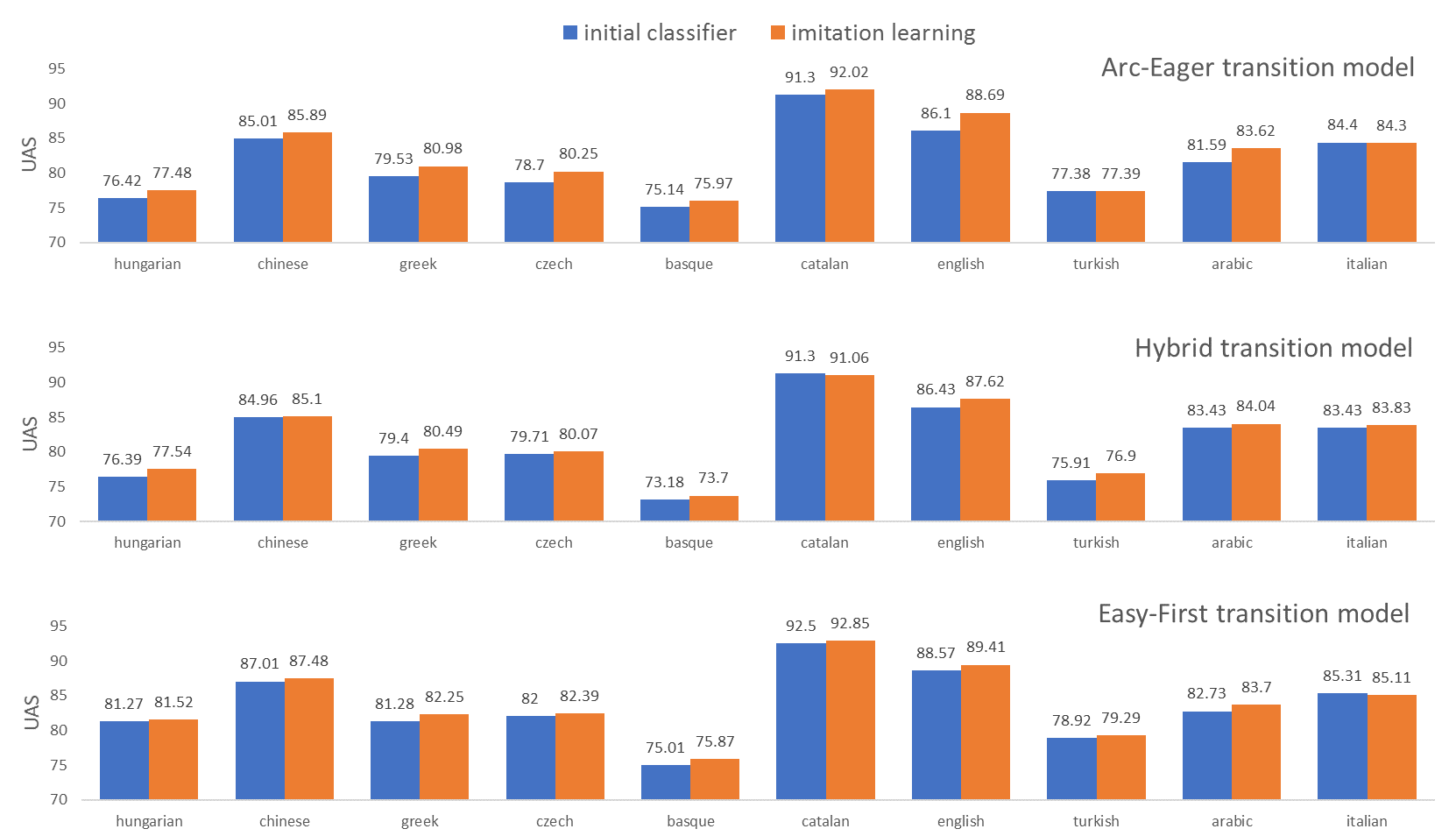### First part recap¶

Imitation Learning

Meta-learning for action-based models:
improve classifier by generating training from demonstrations

### Rollin¶

For each training instance:

• construct an action sequence,
• incrementally transform initial to terminal state.
• Available actions wrt transition system.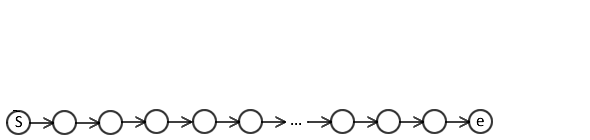### Rollin¶

For each training instance:

• construct an action sequence,
• incrementally transform initial to terminal state.
• Available actions wrt transition system.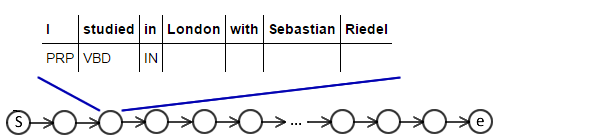### Rollin¶

Can rollin with classifier, expert policy, or a mix.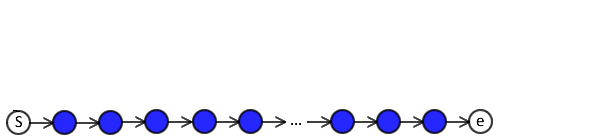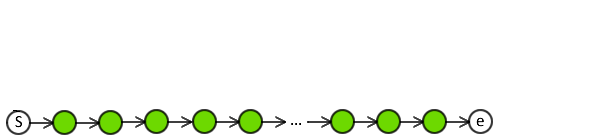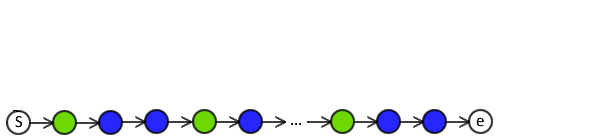### Explore¶

At each timestep:

• explore alternative actions w.r.t transition system.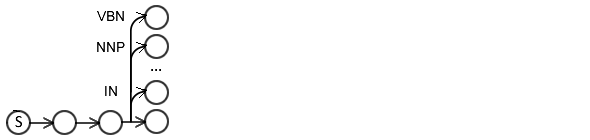### Explore¶

Create a new training instance (feature vector):

• cost alternative actions,
• e.g. DAgger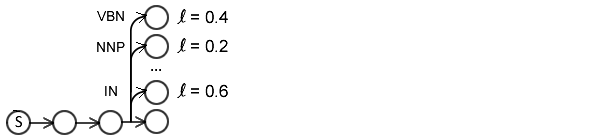### Rollout¶

Or perform rollouts to cost the actions:

• can use non-decomposable loss functions,
• e.g. V-DAgger, SEARN, LOLS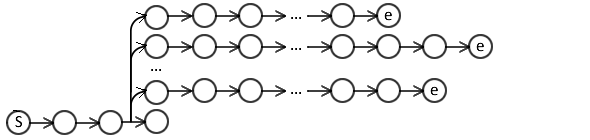### Rollout¶

Can rollout with classifier, expert policy, or a mix.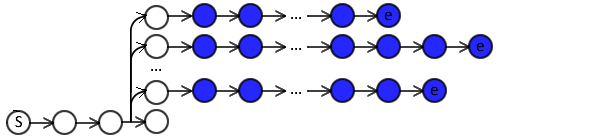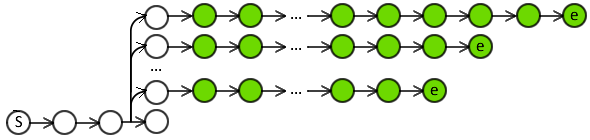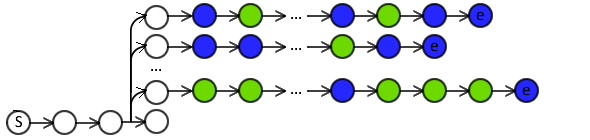### Part 2: NLP Applications and practical advice¶

• Applications:
• dependency parsing
• natural language generation
• semantic parsing
• Practical advice
• making things faster
• debugging

## Applying Imitation Learning to Dependency Parsing

### Dependency parsing¶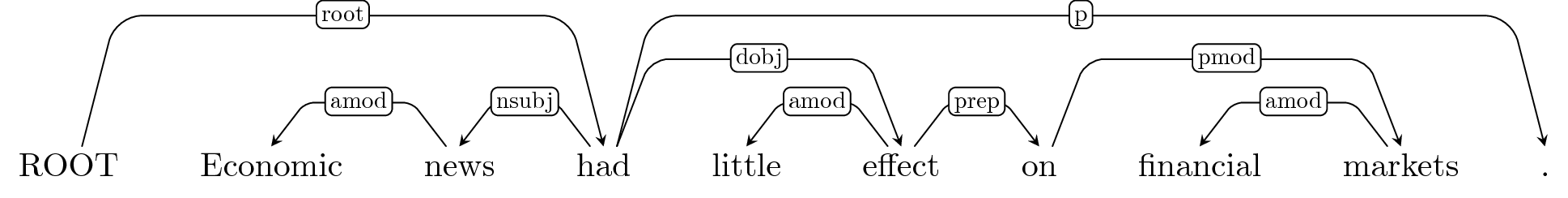To represent the syntax of a sentence as directed labeled arcs between words.

• Labels represent dependencies between words.

### DAgger Reminder¶

\begin{align} & \textbf{Input:} \; D_{train} = \{(\mathbf{x}^1,\mathbf{y}^1)...(\mathbf{x}^M,\mathbf{y}^M)\}, \; \text{expert}\; \pi^{\star}, \; \text{classifier} \; H\\ & \text{set training examples}\; \cal E = \emptyset ,\; \color{red}{\pi^{\star}\; \mathrm{probability}\; \beta=1}\\ & \mathbf{while}\; \text{termination condition not reached}\; \mathbf{do}\\ & \quad \color{red}{\text{set rollin policy} \; \pi^{in} = \beta\pi^{\star} + (1-\beta)H}\\ & \quad \mathbf{for} \; (\mathbf{x},\mathbf{y}) \in D_{train} \; \mathbf{do}\\ & \quad \quad \color{red}{\text{generate trajectory} \; \hat \alpha_1\dots\hat \alpha_T = \pi^{in}(\mathbf{x},\mathbf{y})}\\ & \quad \quad \mathbf{for} \; \hat \alpha_t \in \hat \alpha_1\dots\hat \alpha_T \; \mathbf{do}\\ & \quad \quad \quad \color{red}{\text{ask expert for } \underline{\text{a set of best actions}}\; \{\alpha_{1}^{\star}\dots\alpha_{k}^{\star}\} = \pi^{\star}(\mathbf{x},S_{t-1})} \\ & \quad \quad \quad \text{extract features} \; \mathit{feat}=\phi(\mathbf{x},S_{t-1}) \\ & \quad \quad \quad \cal E = \cal E \cup (\mathit{feat},\alpha^{\star})\\ & \quad \text{learn}\; H \; \text{from}\; \cal E\\ & \quad \color{red}{\text{decrease} \; \beta}\\ \end{align}

To apply Imitation Learning on any task, we need to define:

• Transition
• Loss function
• Expert policy

### Transition system?¶

We can assume any transition-based system (e.g. Arc-Eager).

State: arcs, stack, and buffer.

Action space:
Shift, Reduce, Arc-Left, and Arc-Right.</span>

• Arc-Left / Arc-Right combine with arc labels,
• but limited #labels in dependency parsing (~50).

The length of the transition sequence is variable.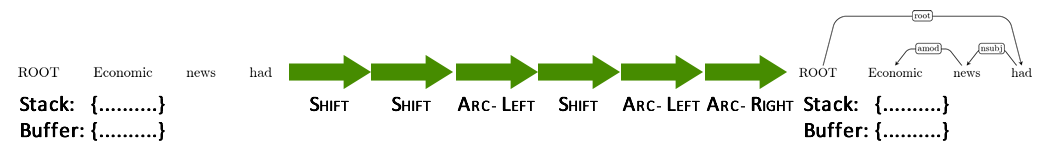Shift -> Shift -> Arc-Right -> Shift -> ... -> Arc-Left

• Bounded (but not fixed) by length of sentence.
• In what task would it be fixed? POS tagging!

### ...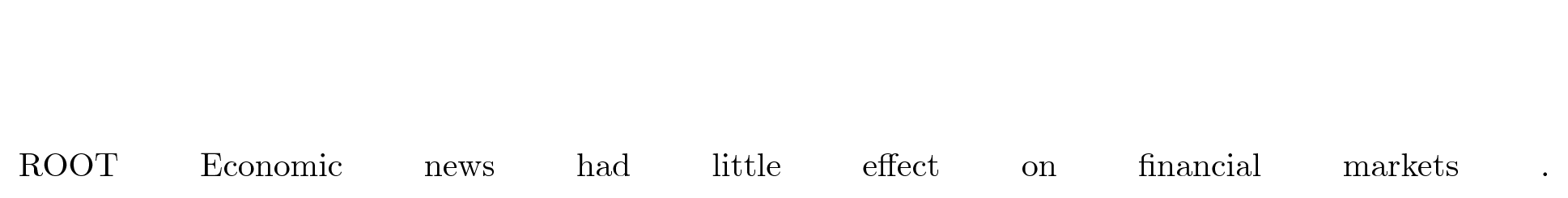Stack: -
Buffer: ROOT, 'economic', 'news', 'had', 'little', 'effect', 'on', 'financial', 'markets', '.'

### ShiftStack: ROOT
Buffer: 'economic', 'news', 'had', 'little', 'effect', 'on', 'financial', 'markets', '.' </font>

### ShiftStack: ROOT, 'economic'
Buffer: 'news', 'had', 'little', 'effect', 'on', 'financial', 'markets', '.' </font>

### Arc-Left: amod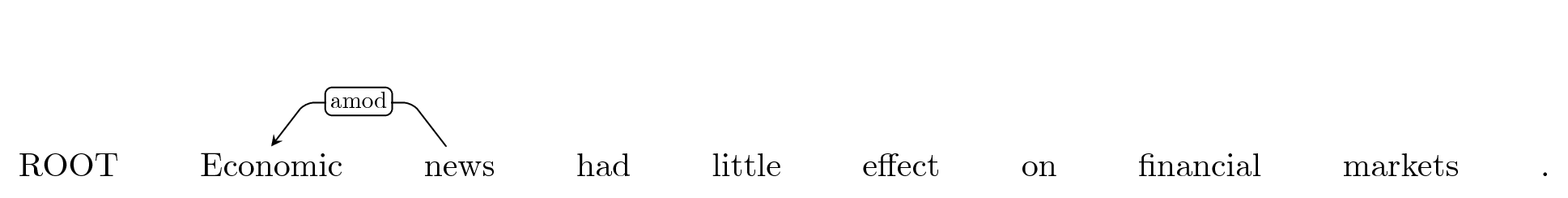Stack: ROOT
Buffer: 'news', 'had', 'little', 'effect', 'on', 'financial', 'markets', '.'

### ShiftStack: ROOT, 'news'
Buffer: 'had', 'little', 'effect', 'on', 'financial', 'markets', '.' </font>

### Arc-Left: nsubj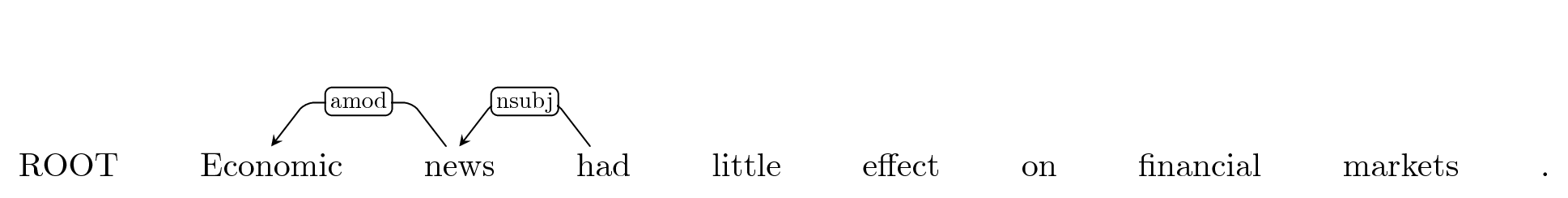Stack: ROOT
Buffer: 'had', 'little', 'effect', 'on', 'financial', 'markets', '.'

### and so onStack: ROOT
Buffer: -

### Loss function?¶

Hamming loss: given predicted arcs, how many parents and labels were incorrectly predicted?

• Directly corresponds to attachment score metrics used to evaluate dependency parsers.
• Costs dependencies, not actions directly.
• Decomposable? Not with this transition model! Cannot score Shift independent of Arc-Right!

### Expert policy?¶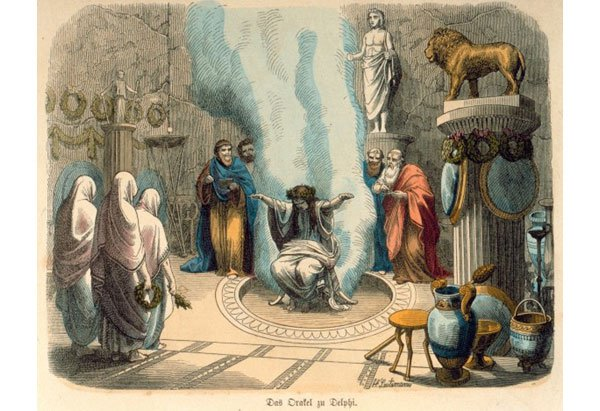Returns the best action at the current state by looking at the gold standard assuming future actions are also optimal:

$$\alpha^{\star}=\pi^{\star}(S_t, \mathbf{y}) = \mathop{\arg \min}_{\alpha \in {\cal A}} L(S_t(\alpha,\pi^{\star}),\mathbf{y})$$

### How do we make an expert policy?¶

We can derive a static transition sequence from initial to terminal state using the golden standard.

• Static expert policy.Static expert does not take previous actions into account.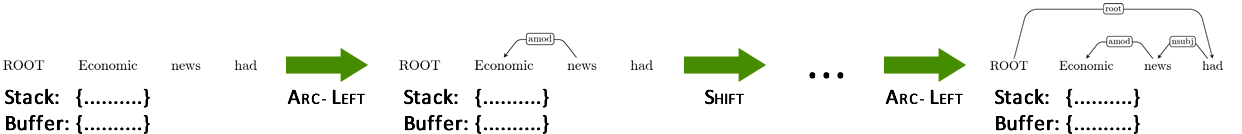Static expert has not encountered this state before.

• Cannot know which is the optimal action.
• May default to an action (e.g. Shift).

A static expert may be sufficient for tasks where we do not care whether the previous actions were optimal.

• What would such a task be? POS tagging.

### Rollin mistakes in dependency parsing¶

Let's assume that we rollin using the classifier.

### Arc-Right: iobj ?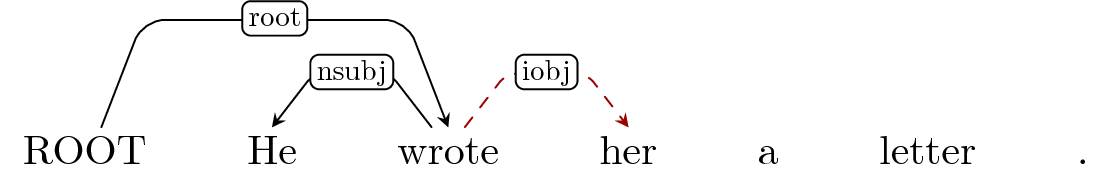Stack: 'wrote'
Buffer: 'her', 'a', 'letter', '.'

### Shift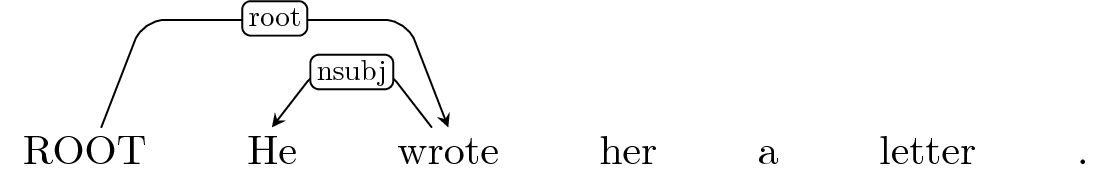Stack: 'wrote', 'her'
Buffer: 'a', 'letter', '.' </font>

### Default: ShiftStack: 'wrote', 'her', 'a'
Buffer: 'letter', '.' </font>

### Arc-Left: det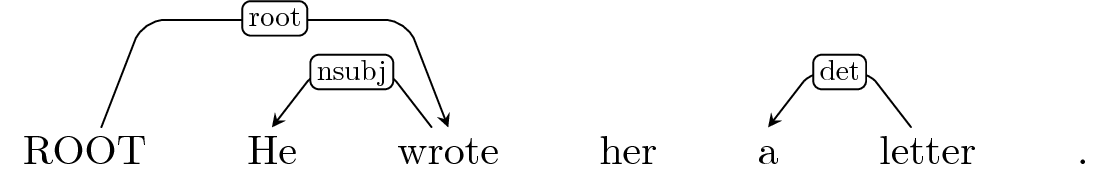Stack: 'wrote', 'her'
Buffer: 'letter', '.'

### Default: ShiftStack: 'wrote', 'her', 'letter'
Buffer: '.' </font>

### Default: ShiftStack: 'wrote', 'her', 'letter', '.'
Buffer: - </font>

Static expert policy cannot recover from errors in the rollin.

### Also, what if there are multiple correct transitions?¶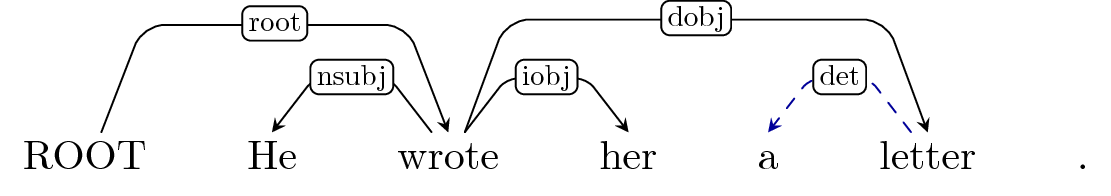Stack: 'her'
Buffer: 'a', 'letter', '.'

Two possible actions: Reduce 'her' / Shift 'a'

Static expert policy arbitarilly choses.

• But chosing any one action indirectly labels the alternative actions as incorrect!
• Leads to noise in the training signal.

### Dynamic expert policy¶

Determines best action, by considering the previous actions.

• Can recover from errors.
• Allows for multiple optimal actions at each time-step.

### Reachable terminal state¶

Reachable terminal state:

• Can be reached through a sequence of expert actions $\alpha_1^{\star}\dots \alpha_T^{\star}$, and
• no further actions can be taken at that state.
• For an optimal reachable terminal state, $L(S_{final}, \mathbf{y}) = 0$.

### How does a dynamic expert policy work?¶

For each possible action at a time-step:

• ...

### ...Stack: 'wrote', 'her'
Buffer: 'letter', '.'

### How does a dynamic expert policy work?¶

For each possible action at a time-step:

• Arc-Left? Arc-Right? Reduce? Shift?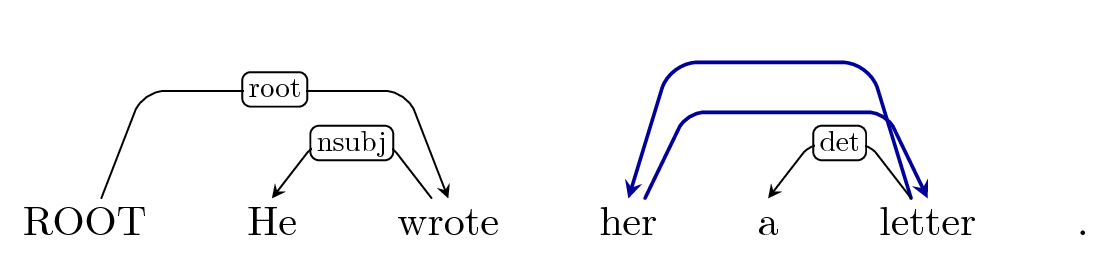Stack: 'wrote', 'her'
Buffer: 'letter', '.'

### How does a dynamic expert policy work?¶

For each possible action at a time-step:

• Arc-Left? Arc-Right? Reduce? Shift?

• Determine the reachable terminal state.
• Use full rollout with expert?

### Arc-Left: det?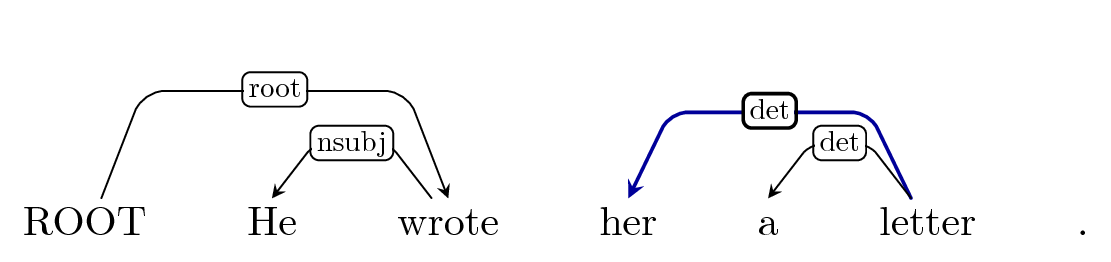Stack: 'wrote',
Buffer: 'letter', '.'

### Arc-Right: dobj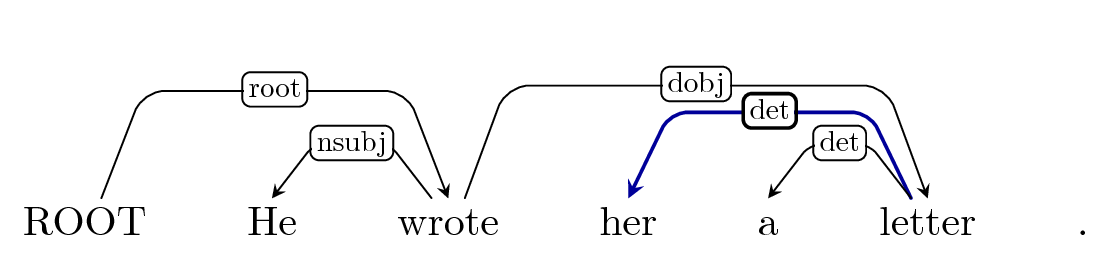Stack: 'wrote',
Buffer: '.'

### Arc-Right: p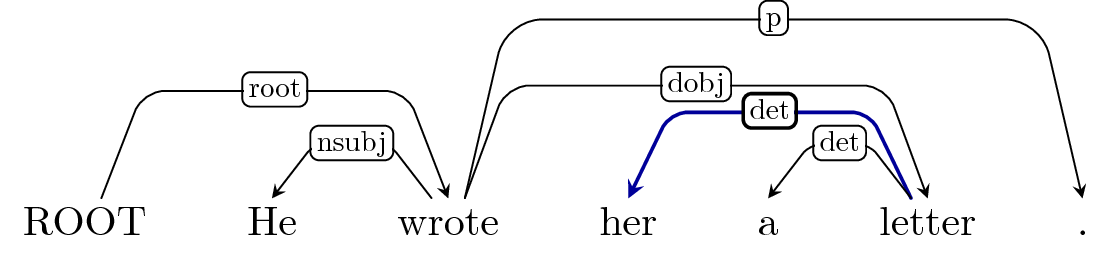Stack: 'wrote',
Buffer: -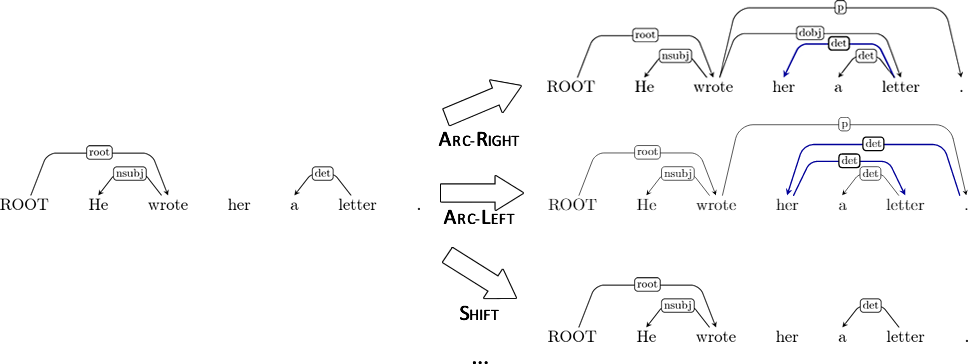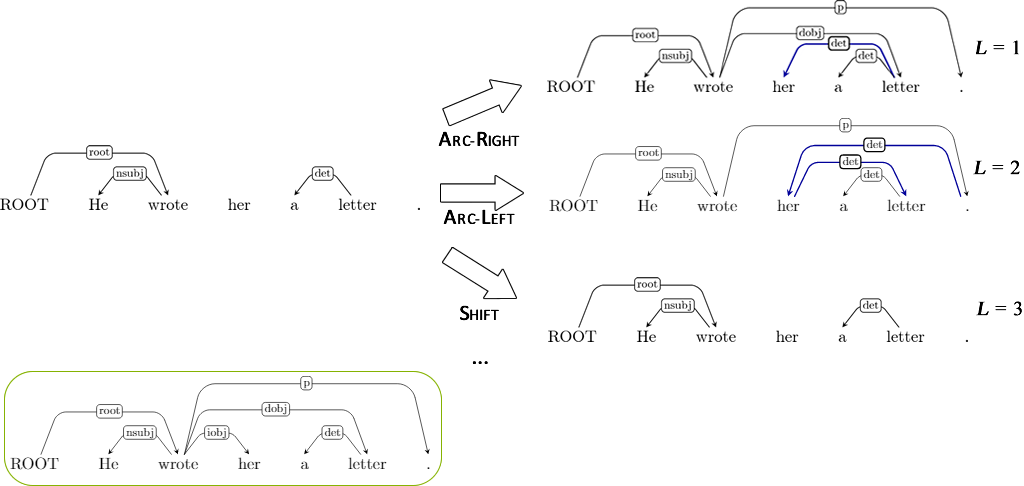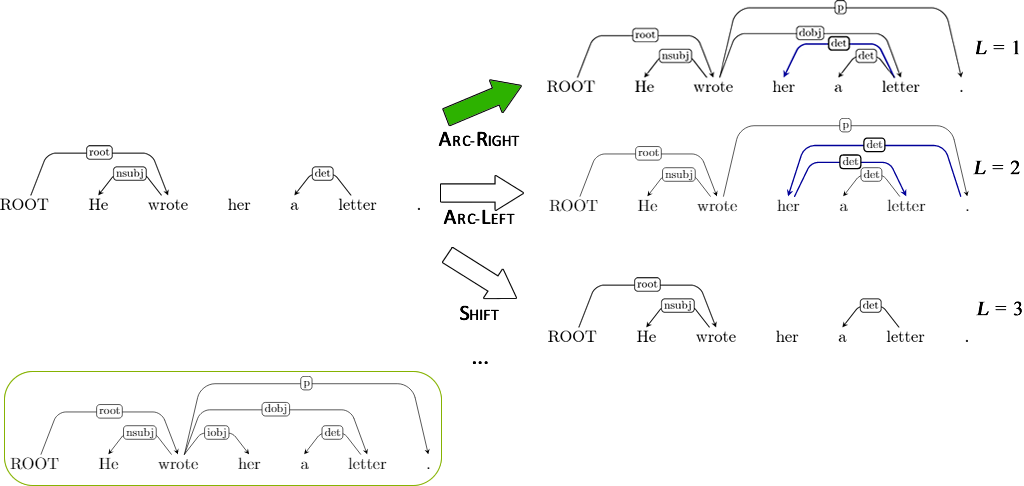### Full rollouts may be expensive¶

Instead of full expert rollout, we may use heuristics!

• i.e. can we estimate how an action will effect the reachable terminal state?

### Arc-Right: det?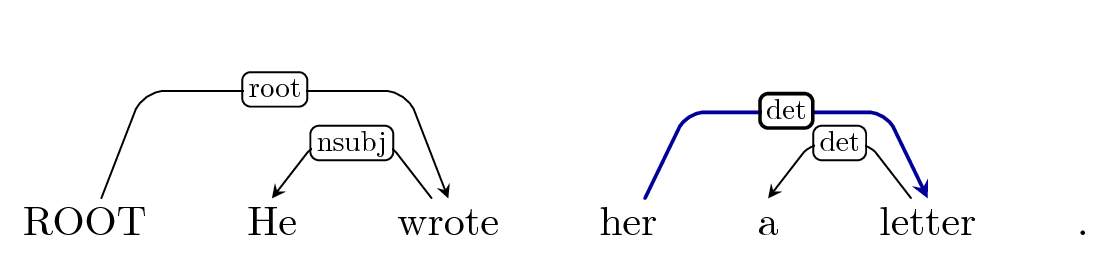Stack: 'wrote', 'her'
Buffer: '.'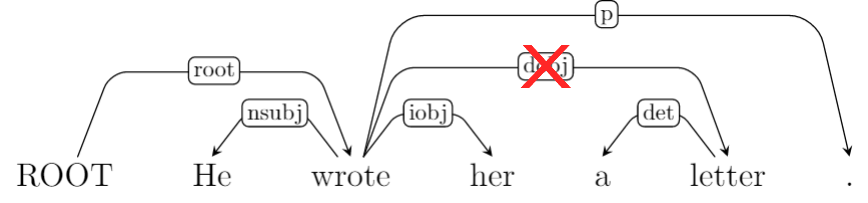### Results

##### [Goldberg and Nivre 2012](http://www.aclweb.org/anthology/C12-1059)